### Home > CALC > Chapter 12 > Lesson 12.2.3 > Problem12-90

12-90.
1. Examine the integral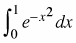. Homework Help ✎

1. Explain why this integral cannot be evaluated exactly using any of our integration tools.

2. Estimate, using a Riemann sum with 5 left-endpoint rectangles.

3. Use a substitution in the expansion of f(x) = ex to get a 6th degree polynomial approximation of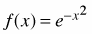.

4. Estimateusing the polynomial of part (c).

5. How could we have improved our answers to parts (b) and (d)?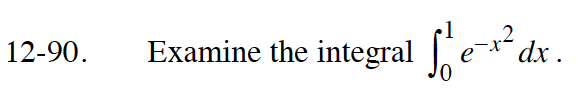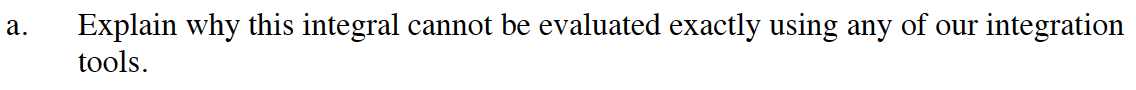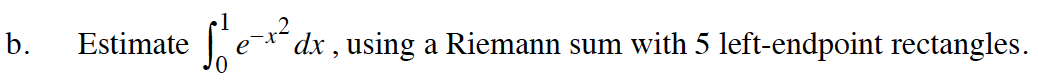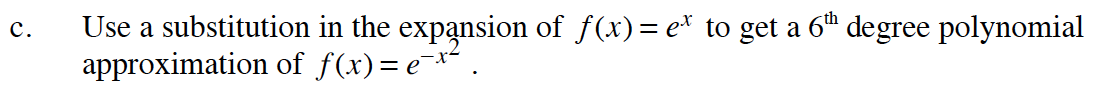$e^x=1+x+\frac{x^2}{2!}+\frac{x^3}{3!}+...$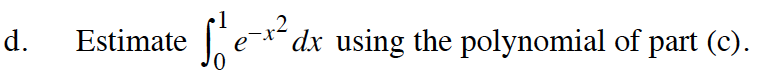$\int_0^1\Big(1-x^2+\frac{x^4}{2}-\frac{x^6}{6}\Big)dx$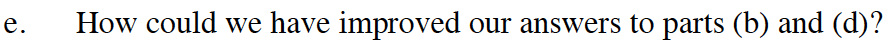Use more ________.